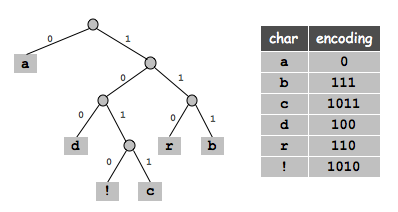# Huffman Text Encrypter

Designed a technique for encrypting data using C++ with the help of Huffman’s Algorithm. Reads the data from file in system and Command Line Interface displays the encoded strings. It takes your text file as input.

## What is huffman encoding?

Huffman enoding is an approach to text compression. In computer science and information theory, it is one of many lossless data compression algorithms. It is a statistical compression method that converts characters into variable length bit strings and produces a preﬁx code. Most frequently occurring characters are converted to shortest bit strings; least frequent, the longest.

## Technique

In Huffman encoding, the complete set of codes can be represented as a binary tree, known as a Huffman tree. This Huffman tree is also a enoding tree i.e. a full binary tree in which each leaf is an encoded symbol and the path from the root to a leaf is its code word. By convention, bit ‘0′ represents following the left child and bit ‘1’ represents following the right child. One code bit represents each level. Thus more frequent characters are near the root and are coded with few bits, and rare characters are far from the root and are coded with many bits.View Github